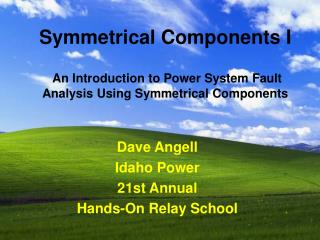DownloadDownload PresentationDave Angell Idaho Power 21st Annual Hands-On Relay School

# Dave Angell Idaho Power 21st Annual Hands-On Relay School

Download Presentation## Dave Angell Idaho Power 21st Annual Hands-On Relay School

- - - - - - - - - - - - - - - - - - - - - - - - - - - E N D - - - - - - - - - - - - - - - - - - - - - - - - - - -
##### Presentation Transcript

1. Symmetrical Components IAn Introduction to Power System Fault Analysis Using Symmetrical Components Dave Angell Idaho Power 21st Annual Hands-On Relay School

2. What Type of Fault?

3. What Type of Fault?

4. What Type of Fault?

5. What Type of Fault?

6. What Type of Fault?

7. What Type of Fault?

8. What Type of Fault?

9. Basic Course Topics • Terminology • Phasors • Equations • Fault Analysis Examples • Calculations

10. Unbalanced Fault

11. Symmetrical Component Phasors • The unbalanced three phase system can be transformed into three balanced phasors. • Positive Sequence • Negative Sequence • Zero Sequence

12. Positive Phase Sequence (ABC)

13. Positive Phase Sequence • Each have the same magnitude. • Each positive sequence voltage or current quantity is displaced 120° from one another.

14. Positive Phase Sequence • The positive sequence quantities have a-b-c, counter clock-wise, phase rotation.

15. Reverse Phase Sequence (ACB)

16. Negative Phase Sequence • Each have the same magnitude. • Each negative sequence voltage or current quantity is displaced 120° from one another.

17. Negative Phase Sequence • The negative sequence quantities have a-c-b, counter clock-wise, phase rotation.

18. Zero Phase Sequence

19. Zero Phase Sequence • Each zero sequence quantity has the same magnitude. • All three phasors with no angular displacement between them, all in phase.

20. Symmetrical Components Equations • Each phase quantity is equal to the sum of its symmetrical phasors. • Va = Va0 + Va1+Va2 • Vb = Vb0 + Vb1 +Vb2 • Vc = Vc0 + Vc1 +Vc2 • The common form of the equations are written in a-phase terms.

21. The a Operator • Used to shift the a-phase terms to coincide with the b and c-phase • Shorthand to indicate 120° rotation. • Similar to the j operator of 90°.

22. Rotation of the a Operator • 120° counter clock-wise rotation. • A vector multiplied by 1 /120° results in the same magnitude rotated 120°.

23. Rotation of the a2 Operator • 240° counter clock-wise rotation. • A vector multiplied by 1 /240° results in the same magnitude rotated 240°.

24. B-Phase Zero Sequence • We replace the Vb sequence terms by Va sequence terms shifted by the a operator. • Vb0 = Va0

25. B-Phase Positive Sequence • We replace the Vb sequence terms by Va sequence terms shifted by the a operator • Vb1 = a2Va1

26. B-Phase Negative Sequence • We replace the Vb sequence terms by Va sequence terms shifted by the a operator • Vb2 = aVa2

27. C-Phase Zero Sequence • We replace the Vc sequence terms by Va sequence terms shifted by the a operator. • Vc0 = Va0

28. C-Phase Positive Sequence • We replace the Vc sequence terms by Va sequence terms shifted by the a operator • Vc1 = aVa1

29. C-Phase Negative Sequence • We replace the Vc sequence terms by Va sequence terms shifted by the a operator • Vc2 = a2Va2

30. What have we produced? • Va = Va0+ Va1 + Va2 • Vb = Va0 + a2Va1 + aVa2 • Vc = Va0 + aVa1 + a2Va2

31. Symmetrical Components Equations • Analysis • To find out of the amount of the components • Synthesis • The combining of the component elements into a single, unified entity

32. Symmetrical Components Synthesis Equations • Va = Va0+ Va1 + Va2 • Vb = Va0 + a2Va1 + aVa2 • Vc = Va0 + aVa1 + a2Va2

33. Symmetrical Components Analysis Equations • Va0 = 1/3 ( Va + Vb + Vc) • Va1= 1/3(Va + aVb + a2Vc) • Va2= 1/3 (Va + a2Vb + aVc)

34. Symmetrical Components Analysis Equations - 1/3 ?? • Where does the 1/3 come from? • Va1= 1/3(Va + aVb + a2Vc) • Va = Va0 + Va1 + Va2 • When balanced

35. Symmetrical Components Analysis Equations - 1/3 ?? • Va1= 1/3(Va + aVb + a2Vc) • Adding the phases

36. Symmetrical Components Analysis Equations - 1/3 ?? • Va1= 1/3(Va + aVb + a2Vc) • Adding the phases yields

37. Symmetrical Components Analysis Equations - 1/3 ?? • Va1= 1/3(Va + aVb + a2Vc) • Adding the phases yields 3 Va. • Divide by the 3 and now Va = Va1

38. Example VectorsAn Unbalanced Voltage • Va = 13.4 /0° • Vb = 59.6 /-104° • Vc = 59.6 /104°

39. Analysis Results in These Sequence Quantities • Va0 = -5.4 • Va1 = 42.9 • Va2 = -24.1

40. Synthesize by Summing the Positive, Negative and …

41. Zero Sequences

42. Three phase fault Positive Phase to phase fault Positive Negative Phase to ground fault Positive Negative Zero Symmetrical Components Present During Shunt Faults

43. Symmetrical Component Review of Faults Types • Let’s return to the example fault reports and view the sequence quantities present

44. Three Phase Fault, Right?

45. A Symmetrical Component View of an Three-Phase Fault

46. A to Ground Fault, Okay?

47. A Symmetrical Component View of an A-Phase to Ground Fault

48. Single Line to Ground Fault • Voltage • Negative and zero sequence 180 out of phase with positive sequence • Current • All sequence are in phase

49. A to B Fault, Easy?